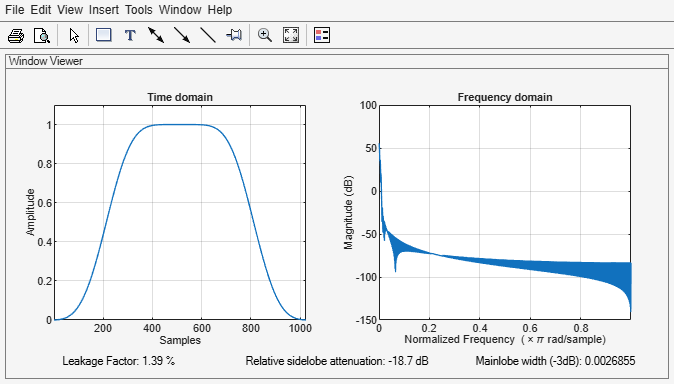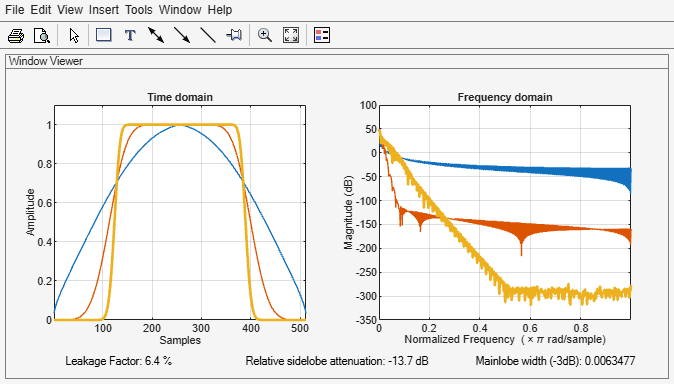kbdwin

Kaiser-Bessel-derived window

Description

example

wdw = kbdwin(N) returns an N-point Kaiser-Bessel-derived (KBD) window.

example

wdw = kbdwin(N,Beta) specifies the tuning parameter, Beta.

Examples

collapse all

Create a 1024-point Kaiser-Bessel-derived (KBD) window. Visualize the KBD window in the time and frequency domains using wvtool.

wdw = kbdwin(1024);
wvtool(wdw)Create three 512-point KBD windows, with Beta set to 1, 10, and 100. Display the windows for comparison using wvtool.

N = 512;
beta1 = kbdwin(N,1);
beta10 = kbdwin(N,10);
beta100 = kbdwin(N,100);

wvtool(beta1,beta10,beta100)Input Arguments

collapse all

Number of points in the KBD window, specified as an even positive integer scalar.

Data Types: single | double

Tuning parameter, specified as a nonnegative real scalar. If unspecified, Beta defaults to 5.

Data Types: single | double

Output Arguments

collapse all

Kaiser-Bessel-derived window, returned as an N-point column vector.

Algorithms

The coefficients of a Kaiser-Bessel-derived window are computed using the equation:

where w is a Kaiser window designed using the kaiser function:

w = kaiser(N/2+1,Beta*pi)
where N is the number of points in the KBD window and Beta is the tuning parameter.

 Bosi, Marina, and Richard E. Goldberg. Introduction to Digital Audio Coding and Standards. Dordrecht: Kluwer, 2003.

Audio Toolbox DocumentationGet trial now